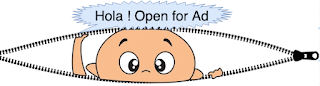## Latest Posts

Size of binary tree is total number of nodes in the given binary tree.For the following binary tree size is 11. In this post we have discussed both recursive and iterative approach to find size of binary tree.

Sample code for searching  an element in binary tree in Java - recursive approach
Algorithm:-
1. Traverse given binary tree and increment size by 1 for each node.
2. Call recursive method for each left and right child and repeat step 1 and step 2.
3. While returning from leaf to root, size is added and returned.
Time and space complexity :- Time complexity and space complexity is order of O(n).
`/* Recursive approach to find total number of nodes */public int findSizeOfBinaryTreeRecursive(Node root) { int size = 0; if (root == null) {  return 0; } /*  * for each node increment size and call function recursively for left  * and right child.  */ size = 1 + findSizeOfBinaryTreeRecursive(root.leftChild)   + findSizeOfBinaryTreeRecursive(root.rightChild); return size;}`

Sample code for searching  an element in binary tree in Java - iterative approach
Algorithm:-
1. Do level order traversal and increment size for each node.

2. Once queue is empty, return size of binary tree.
Note:-
We can also use other iterative traversals(preorder, postorder and inorder) for calculating size of tree.
Time and space complexity :-
Time complexity and space complexity is order of O(n).

`/* Iterative approach to find total number of nodes-level order traversal */public int findSizeOfBinaryTreeIterative(Node root) { Node temp; int size = 0; Queue<Node> queue = new ArrayDeque<>(); queue.add(root); while (!queue.isEmpty()) {  temp = queue.remove();  size++; // Increment size on each pop up operation  Node lc = temp.leftChild;  Node rc = temp.rightChild;  if (lc != null) {   queue.add(lc);  }  if (rc != null) {   queue.add(rc);  } } return size;}`

#### Complete sample program -finding size of binary tree in Java (Iterative and Recursive)

`package com.devinline.trees;import java.util.ArrayDeque;import java.util.Queue;public class SizeOfBinaryTree { /* Iterative approach to find total number of nodes-level order traversal */ public int findSizeOfBinaryTreeIterative(Node root) {  Node temp;  int size = 0;  Queue<Node> queue = new ArrayDeque<>();  queue.add(root);  while (!queue.isEmpty()) {   temp = queue.remove();   size++; // Increment size on each pop up operation   Node lc = temp.leftChild;   Node rc = temp.rightChild;   if (lc != null) {    queue.add(lc);   }   if (rc != null) {    queue.add(rc);   }  }  return size; } /* Recursive approach to find total number of nodes */ public int findSizeOfBinaryTreeRecursive(Node root) {  int size = 0;  if (root == null) {   return 0;  }  /*   * for each node increment size and call function recursively for left   * and right child.   */  size = 1 + findSizeOfBinaryTreeRecursive(root.leftChild)    + findSizeOfBinaryTreeRecursive(root.rightChild);  return size; } public static void main(String[] args) {  SizeOfBinaryTree sizeObj = new SizeOfBinaryTree();  Node root = sizeObj.new BinaryTree().createTree();  int size = sizeObj.findSizeOfBinaryTreeIterative(root);  System.out.println("Iterative -size of binary tree :- " + size);  size = sizeObj.findSizeOfBinaryTreeRecursive(root);  System.out.println("Recursive size of binary tree :-  " + size); } class Node {  private int data;  private Node leftChild;  private Node rightChild;  public Node(int data) {   this.data = data;   leftChild = null;   rightChild = null;  }  public int getData() {   return data;  }  public void setData(int data) {   this.data = data;  }  public Node getLeftChild() {   return leftChild;  }  public void setLeftChild(Node leftChild) {   this.leftChild = leftChild;  }  public Node getRightChild() {   return rightChild;  }  public void setRightChild(Node rightChild) {   this.rightChild = rightChild;  } } class BinaryTree {  Node root;  public BinaryTree() {   root = null;  }  public Node createTree() {   if (root == null) {    root = new Node(12);   }   root.setLeftChild(new Node(23));   root.setRightChild(new Node(18));   root.getLeftChild().setLeftChild(new Node(11));   root.getLeftChild().setRightChild(new Node(43));   root.getRightChild().setRightChild(new Node(27));   root.getRightChild().setLeftChild(new Node(12));   root.getLeftChild().getLeftChild().setLeftChild(new Node(56));   root.getLeftChild().getLeftChild().setRightChild(new Node(78));   root.getRightChild().getLeftChild().setRightChild(new Node(98));   root.getRightChild().getLeftChild().setLeftChild(new Node(32));   return root;  } }}`
=======Sample output========
Iterative -size of binary tree :-   11
Recursive size of binary tree :-  11
=========================

#### 1 comment:

1.